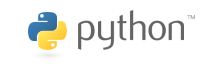# Data analysis: Pandas and Seaborn¶

## Yoav Ram¶

Pandas is a very strong library for manipulating large and complex datasets using a new data structure, the data frame, which models a table of data. Pandas helps to close the gap between Python and R for data analysis and statistical computing.

Pandas data frames address three deficiencies of NumPy arrays:

• data frame hold heterogenous data; each column can have its own numpy.dtype,
• the axes of a data frame are labeled with column names and row indices,
• and, they account for missing values which this is not directly supported by arrays.

Data frames are extremely useful for data manipulation. They provide a large range of operations such as filter, join, and group-by aggregation, as well as plotting.

In :
import numpy as np
import pandas as pd
print('Pandas version:', pd.__version__)

Pandas version: 0.25.3


# Statistical Analysis of Life History Traits¶

We will analyze animal life-history data from AnAge.

In :
data = pd.read_csv('../data/anage_data.txt', sep='\t') # lots of other pd.read_... functions
print(type(data))
print(data.shape)

<class 'pandas.core.frame.DataFrame'>
(4219, 31)


Pandas holds data in DataFrame (similar to R). DataFrame have a single row per observation (in contrast to the previous exercise in which each table cell was one observation), and each column has a single variable. Variables can be numbers or strings.

The head method gives us the 5 first rows of the data frame.

In :
data.head()

Out:
HAGRID Kingdom Phylum Class Order Family Genus Species Common name Female maturity (days) ... Source Specimen origin Sample size Data quality IMR (per yr) MRDT (yrs) Metabolic rate (W) Body mass (g) Temperature (K) References
0 3 Animalia Arthropoda Branchiopoda Diplostraca Daphniidae Daphnia pulicaria Daphnia NaN ... NaN unknown medium acceptable NaN NaN NaN NaN NaN 1294,1295,1296
1 5 Animalia Arthropoda Insecta Diptera Drosophilidae Drosophila melanogaster Fruit fly 7.0 ... NaN captivity large acceptable 0.05 0.04 NaN NaN NaN 2,20,32,47,53,68,69,240,241,242,243,274,602,98...
2 6 Animalia Arthropoda Insecta Hymenoptera Apidae Apis mellifera Honey bee NaN ... 812 unknown medium acceptable NaN NaN NaN NaN NaN 63,407,408,741,805,806,808,812,815,828,830,831...
3 8 Animalia Arthropoda Insecta Hymenoptera Formicidae Cardiocondyla obscurior Cardiocondyla obscurior NaN ... 1293 captivity medium acceptable NaN NaN NaN NaN NaN 1293
4 9 Animalia Arthropoda Insecta Hymenoptera Formicidae Lasius niger Black garden ant NaN ... 411 unknown medium acceptable NaN NaN NaN NaN NaN 411,813,814

5 rows × 31 columns

DataFrame has many of the features of numpy.ndarray - it also has a shape and various statistical methods (max, mean etc.). However, DataFrame allows richer indexing. For example, let's browse our data for species that have body mass greater than 300 kg. First we will a create new column (Series object) that tells us if a row is a large animal row or not:

In :
large_index = data['Body mass (g)'] > 300 * 1000 # 300 kg

Out:
0    False
1    False
2    False
3    False
4    False
Name: Body mass (g), dtype: bool

Now, we slice our data with this boolean index. The iterrows method let's us iterate over the rows of the data. For each row we get both the row as a Series object (similar to dict for our use) and the row number as an int (this is similar to the use of enumerate on lists and strings).

In :
large_data = data[large_index]
for i, row in large_data.iterrows():
print(row['Common name'], row['Body mass (g)']/1000, 'kg')

Domestic cattle 347.0 kg
Dromedary 407.0 kg
Moose 325.0 kg
Asiatic elephant 3672.0 kg
West Indian manatee 450.0 kg


So... a Dromedary is the single-humped camel.Let's continue with small and medium animals - we filter out anything that doesn't have body mass of less than 300 kg.

In :
data = data[data['Body mass (g)'] <  300 * 1000]


For starters, let's plot a scatter of body mass vs. metabolic rate. Because we work with pandas, we can do that with the plot method of DataFrame, specifying the columns for x and y and a plotting style (without the style we would get a line plot which makes no sense here).

You can change %matplotlib inline to %matplotlib widget to get interactive plotting -- if this causes errors, just stay with inline, as the widget feature is new and may require to update some packages.

In :
%matplotlib inline
import matplotlib.pyplot as plt

In :
data.plot.scatter(x='Body mass (g)', y='Metabolic rate (W)', legend=False)
plt.ylabel('Metabolic rate (W)');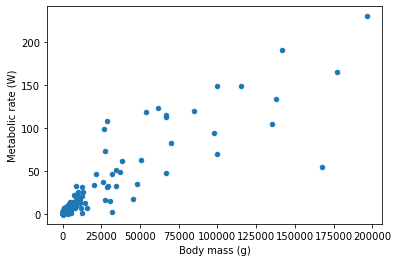If this plot looks funny, you are probably using Pandas with version <0.22; the bug was reported and fixed in version 0.22.

From this plot it seems that

1. there is a correlation between body mass and metabolic rate, and
2. there are many small animals (less than 30 kg) and not many medium animals (between 50 and 300 kg).

Before we continue, I prefer to have mass in kg, let's add a new column:

In :
data['Body mass (kg)'] = data['Body mass (g)'] / 1000


Next, let's check how many records do we have for each Class (as in the taxonomic unit):

In :
class_counts = data['Class'].value_counts()
print(class_counts)

Mammalia    417
Aves        171
Amphibia     18
Reptilia     16
Name: Class, dtype: int64

In :
# plt.figure() # only required if you used %matplotlib widget
class_counts.plot.bar()
plt.ylabel('Num. of species');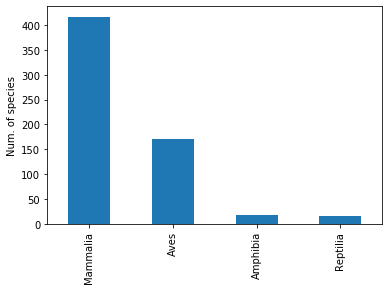So we have lots of mammals and birds, and a few reptiles and amphibians. This is important as amphibian and reptiles could have a different replationship between mass and metabolism because they are cold blooded.

## Exercise: data frames¶

1) Print the number of reptiles are in this dataset, and how many of them are of the genus Python.

Reminder

• Edit cell by double clicking
• Run cell by pressing Shift+Enter
• Get autocompletion by pressing Tab
• Get documentation by pressing Shift+Tab
In :


In :
print("# of reptiles: ", reptiles)
print("# of pythons: ", pythons)

# of reptiles:  16
# of pythons:  2


2) Plot the histogram of the mammal body masses using plot.hist(). Since most mammals are small, the histogram looks better if we plot a cumulative distribution rather then the distribution - we can do this with the cumulative argument. You also need to specify a higher bins argument then the default.

In [ ]:


In :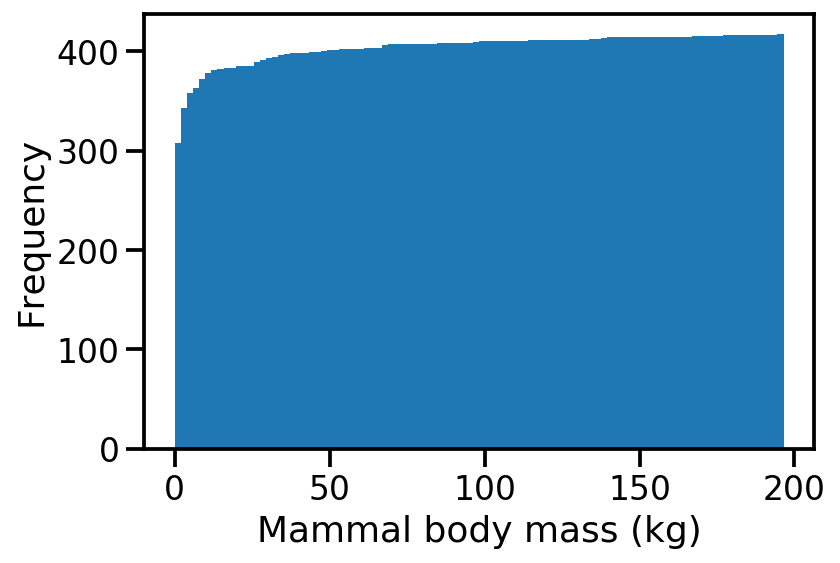# Seaborn¶

Let's do a simple linear regression plot; but let's do it in separate for each Class. We can do this kind of thing with Matplotlib and SciPy, but a very good tool for statistical visualizations is Seaborn.

Seaborn adds on top of Pandas a set of sophisticated statistical visualizations, similar to ggplot2 for R.

In :
import seaborn as sns
sns.set_context("talk")

In :
sns.lmplot(
x='Body mass (kg)',
y='Metabolic rate (W)',
hue='Class',
data=data,
ci=False,
);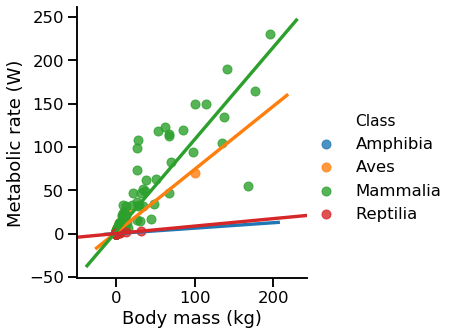• hue means color, but it also causes seaborn to fit a different linear model to each of the Classes.
• ci controls the confidence intervals. I chose False, but setting it to True will show them.

We can see that mammals and birds have a clear correlation between size and metabolism and that it extends over a nice range of mass, so let's stick to mammals; next up we will see which orders of mammals we have.

In :
mammalia = data[data.Class=='Mammalia']
order_counts = mammalia.Order.value_counts()
ax = order_counts.plot.barh()
ax.set(
xlabel='Num. of species',
ylabel='Mammalia order'
)
ax.figure.set_figheight(7)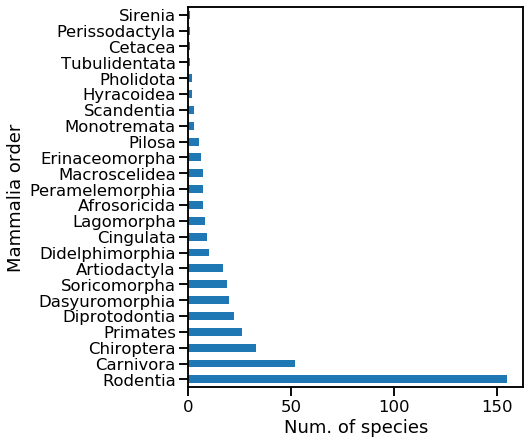You see we have alot of rodents and carnivores, but also a good number of bats (Chiroptera) and primates.

Let's continue with orders that have at least 20 species - this also includes some cool marsupials like Kangaroo, Koala and Taz (Diprotodontia and Dasyuromorphia)

In :
orders = order_counts[order_counts >= 20]
print(orders)
abund_mammalia = mammalia[mammalia.Order.isin(orders.index)]

Rodentia          155
Carnivora          52
Chiroptera         33
Primates           26
Diprotodontia      22
Dasyuromorphia     20
Name: Order, dtype: int64

In :
sns.lmplot(
x='Body mass (kg)',
y='Metabolic rate (W)',
hue='Order',
data=abund_mammalia,
ci=False,
height=8,
aspect=1.3,
line_kws={'lw':2, 'ls':'--'},
scatter_kws={'s':50, 'alpha':0.5}
);
# if you get an error about height not being a keyword, change it to size or update seaborn: conda update seaborn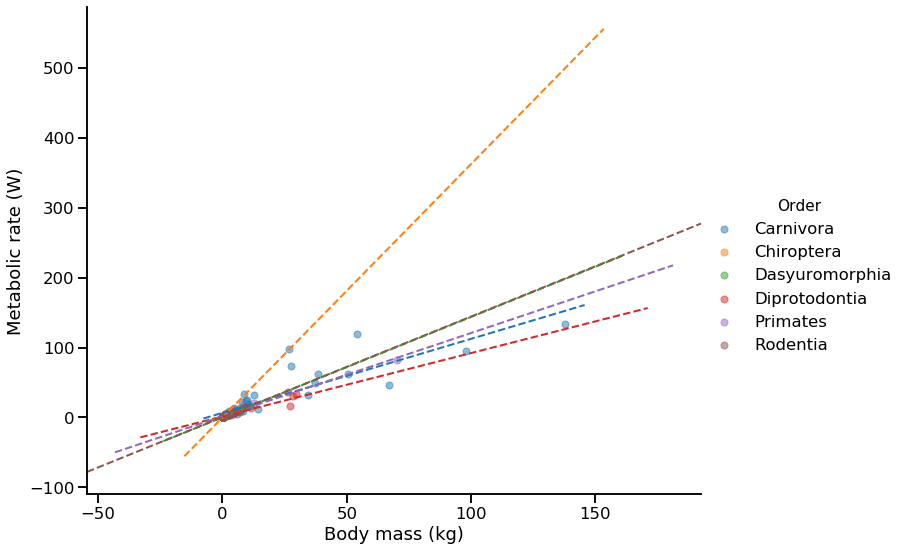Because there is alot of data here I made the lines thinner - this can be done by giving matplotlib keywords as a dictionary to the argument line_kws - and I made the markers bigger but with alpha (transperancy) 0.5 using the scatter_kws argument.

Still ,there's too much data, and part of the problem is that some orders are large (e.g. primates) and some are small (e.g. rodents).

Let's plot a separate regression plot for each order. We do this using the col and row arguments of lmplot, but in general this can be done for any plot using seaborn's FacetGrid function.

In :
sns.lmplot(
x='Body mass (kg)',
y='Metabolic rate (W)',
data=abund_mammalia,
hue='Order',
col='Order',
col_wrap=3,
ci=None,
scatter_kws={'s':40},
sharex=False,
sharey=False
);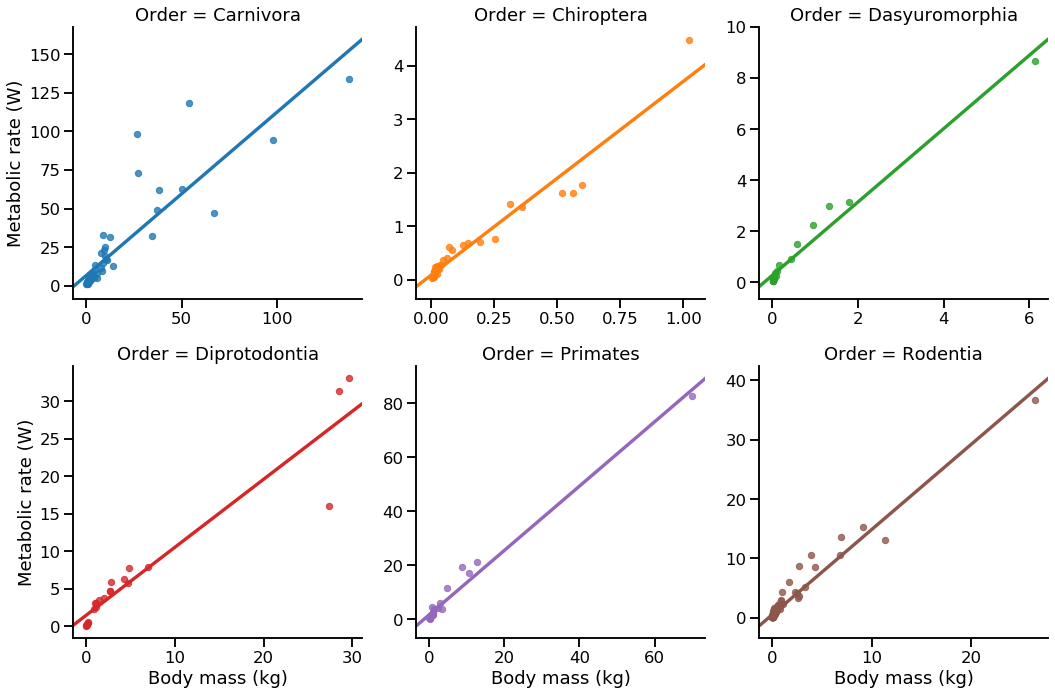We used the sharex=False and sharey=False arguments so that each Order will have a different axis range and so the data is will spread nicely.

# Statistics¶

Lastly, let's do some quick statistics.

First, calculate a summary of the the mammals using describe.

In :
mass = abund_mammalia
mass.describe()

Out:
HAGRID Female maturity (days) Male maturity (days) Gestation/Incubation (days) Weaning (days) Litter/Clutch size Litters/Clutches per year Inter-litter/Interbirth interval Birth weight (g) Weaning weight (g) Adult weight (g) Growth rate (1/days) Maximum longevity (yrs) IMR (per yr) MRDT (yrs) Metabolic rate (W) Body mass (g) Temperature (K) Body mass (kg)
count 308.000000 255.000000 190.000000 279.000000 252.000000 306.000000 250.00000 200.000000 260.000000 169.000000 308.000000 104.000000 259.000000 5.000000 5.000000 308.000000 308.000000 273.000000 308.000000
mean 2341.662338 378.423529 445.689474 62.573477 80.396825 3.340686 1.96900 240.650000 173.817317 1055.666805 5048.303084 0.031044 14.732819 0.014640 2.620000 5.722388 3666.744805 309.793516 3.666745
std 335.937489 412.882713 503.681172 52.988741 85.983514 2.266984 1.36074 179.327688 979.288670 3478.769937 18036.408022 0.024898 11.109210 0.025658 3.395144 16.048335 12815.685721 1.419072 12.815686
min 1722.000000 24.000000 36.000000 12.000000 13.000000 1.000000 0.30000 20.000000 0.004000 2.700000 4.200000 0.000500 2.500000 0.000200 0.300000 0.027000 3.700000 305.150000 0.003700
25% 2027.250000 112.000000 160.750000 25.000000 29.500000 1.500000 1.00000 60.000000 2.327500 15.000000 40.000000 0.014150 6.850000 0.001000 0.300000 0.306500 38.100000 309.050000 0.038100
50% 2477.000000 315.000000 350.000000 37.000000 50.500000 3.000000 1.55000 241.000000 6.685000 50.500000 170.950000 0.024700 12.000000 0.002000 0.500000 0.739500 170.750000 309.850000 0.170750
75% 2620.250000 476.000000 548.000000 89.000000 91.750000 4.775000 2.10000 365.000000 45.000000 500.000000 1500.000000 0.041325 20.500000 0.010000 4.000000 3.578250 1288.500000 310.750000 1.288500
max 2831.000000 4745.000000 5110.000000 280.000000 639.000000 22.200000 10.00000 1095.000000 11000.000000 30000.000000 175000.000000 0.130000 122.500000 0.060000 8.000000 133.859000 137900.000000 313.850000 137.900000

Now lets check if we can significantly say that the body mass of rodents is lower than that of carnivores.

## Exercise: boxplot¶

Plot boxplots of the mammals body mass using Seaborn, which is easier to use (and also makes nicer boxplots) then standard matplotlib boxplot.

In [ ]:


In :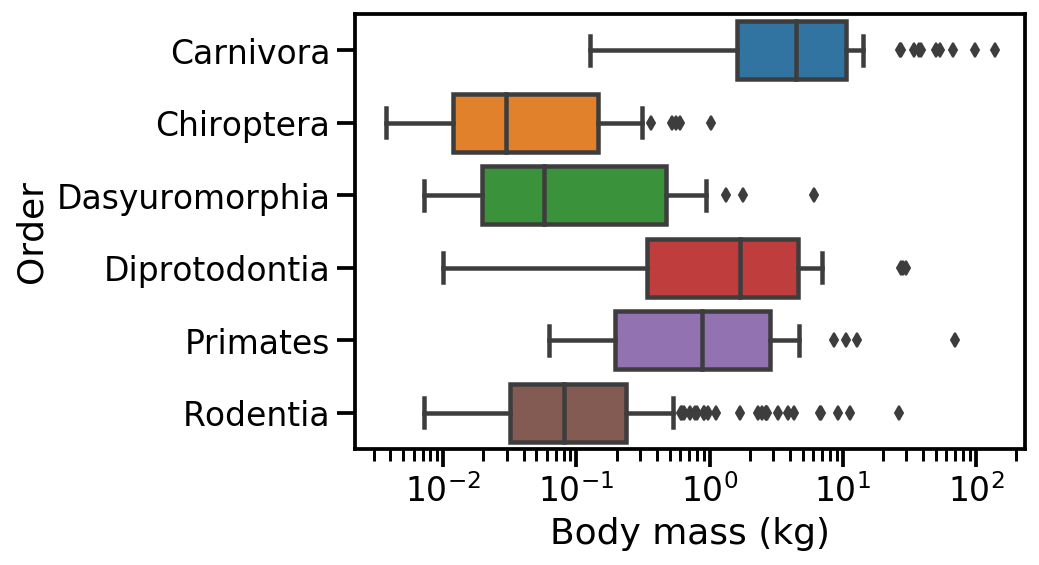Now, we'll use a t-test (implemented in the scipy.stats module) to test the hypothesis that there is no difference in body mass between rodents and carnivores.

• ttest_ind calculates the t-test for the means of two independent samples of scores.
• scipy.stats has many more statistical tests, distributions, etc.
In :
from scipy.stats import ttest_ind

In :
carnivora_mass = abund_mammalia.loc[abund_mammalia['Order']=='Carnivora', 'Body mass (kg)']
rodentia_mass = abund_mammalia.loc[abund_mammalia['Order']=='Rodentia', 'Body mass (kg)']

res = ttest_ind(carnivora_mass, rodentia_mass, equal_var=False)
print("P-value of t-test: {:.2g}".format(res.pvalue))

P-value of t-test: 0.00033


# Colophon¶

This notebook was written by Yoav Ram.

The notebook was written using Python 3.7. Dependencies listed in environment.yml.